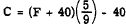PrevIndexNext

# Looping

In this section we see how to have Perl take actions over and over again. With just a few compact statements we can have our programs do many many things for us.
\$i = 1; while (\$i <= 10) { print "\$i\n"; \$i = \$i + 1; }
The 'while' statement is similar in syntax to the 'if' statement. The difference is that it executes the body (between { }) repeatedly until the boolean condition is false. Do you see how the code above works?

What would happen if we didn't add 1 to \$i? Make sure that your while loops eventually end - otherwise you will have an INFINITE LOOP!!! It will run forever. Control-C will kill it.

As with the 'if', curly braces are needed even if there is only one statement inside the body of the while.

The following 'for' statement is exactly equivalent to the above:

for (\$i = 1; \$i <= 10; \$i = \$i + 1) { print "\$i\n"; }
The 'for' is sometimes better than the while because all the elements of loop control are in one place.

## Loop Control Statements

The 'next' keyword will continue the next iteration of the loop - either a 'for' or a 'while'. 'last' will exit the loop immediately - either 'for' or 'while'.
\$i = 1; while (1) { # an infinite loop! yikes! if (\$i > 10) { last; # get out now } if (\$i == 5) { \$i = \$i + 1; # can't forget this! next; # go test the while boolean again } print "\$i\n"; \$i = \$i + 1; }
Sometimes it is clearer to have the boolean be always true (1) and use 'last' to exit the loop when a condition is reached.

Note that when using 'next' in a 'for' loop the flow of control is transfered to the increment part. So this will work:

for (\$i = 0; \$i < 20; ++\$i) { if (10 <= \$i and \$i <= 14) { next; # on to the increment section: ++\$i; } print "\$i\n"; }

## Increment/Decrement and Assignment Operators

All the following have the same effect - the variable is incremented by 1.
\$i = \$i + 1; ++\$i; \$i++; \$i += 1;

Decrementing by one is similar:

--\$i;
These pairs of statements have the same effect:
\$i = \$i * 4; \$i *= 4; \$i += 2; \$i = \$i + 2;
These "assignment operators" are sometimes clearer and easier to type and read. For example:
\$total_count_of_units = \$total_count_of_units + \$n; \$total_count_of_units += \$n;

## Mistyped Variable names and -w

It is easy to misytpe variabel names. Using -w on the perl command line will give warnings about such misteaks and several other helpful things as well.
#!/usr/bin/perl -w
It is a good idea to ALWAYS use -w. You can also (since Perl 5.6.0) use the following within your program:
use warnings;

# Exercises

1. Print a table of Fahrenheit-Centigrade conversion from 32 to 72 in steps of 2 degrees. Leave the range 40-46 out.2. Ask the user for two integers. If the first is greater than the second print an error message and quit. Otherwise, total up the the numbers between (and including) the first and the second and print the sum. For example:
First? 3 Second? 8 Sum: 33
This is because 3 + 4 + 5 + 6 + 7 + 8 = 33.
3. Ask the user for a number between 1 and 10. If they give a number outside that range, print an error message and keep asking. If they don't give a right answer after 10 tries give up on them. If they type a 'q' or a 'Q', quit with no further output at all. If they do eventually give a right answer print the number of times they gave a wrong answer.
4. Choose a secret number between 1 and 100 and ask the user to guess it. Tell them whether they were too high or too low. Keep asking until they get it. If they type '?', tell them what it was.

This statement:

\$n = int(rand 100) + 1;
will assign \$n a random integer between 1 and 100.